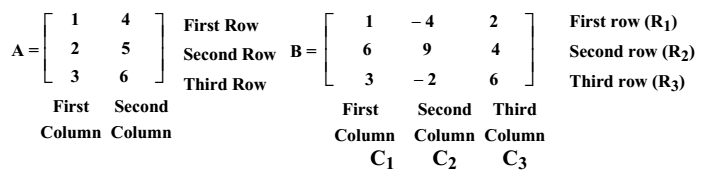# Introduction to Matrix

The term matrix was first introduced by Sylvester in 1850. He defined a matrix to be an arrangement of terms. In 1858, Cayley outlined a matrix algebra defining addition, multiplication, scalar multiplication and inverses.

The purpose of matrices is to provide a kind of mathematical shorthand to help the study of problems represented by the entries. The matrices may represent transformations of co-ordinate spaces or systems of simultaneous linear equations.

### Definition

A matrix is a rectangular array or arrangement of entries or elements displayed in rows and columns put within a square bracket or parenthesis. The  entries or elements may be any kind of numbers (real or complex), polynomials or other expressions. Matrices are denoted by the capital letters like A, B, or C.In a matrix, rows are counted from top to bottom and the columns are counted from left to right.

The horizontal arrangements are known as rows. The vertical arrangements are known as columns.

To identify an entry or an element of a matrix two suffixes are used. The first suffix denotes the row and the second suffix denotes the column in which the element occurs.

For example, the elements of A are

• a11 = 1
• a12 = 4
• a21 = 2
• a22 = 5
• a31 = 3
• a32 = 6